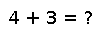# svdfact(A)

svdfact(A, [thin=true]) -> SVD

Compute the Singular Value Decomposition (SVD) of `A` and return an `SVD` object. `U`, `S`, `V` and `Vt` can be obtained from the factorization `F` with `F[:U]`, `F[:S]`, `F[:V]` and `F[:Vt]`, such that `A = U*diagm(S)*Vt`. If `thin` is `true`, an economy mode decomposition is returned. The algorithm produces `Vt` and hence `Vt` is more efficient to extract than `V`. The default is to produce a thin decomposition.

## Examples

Checking you are not a robot: